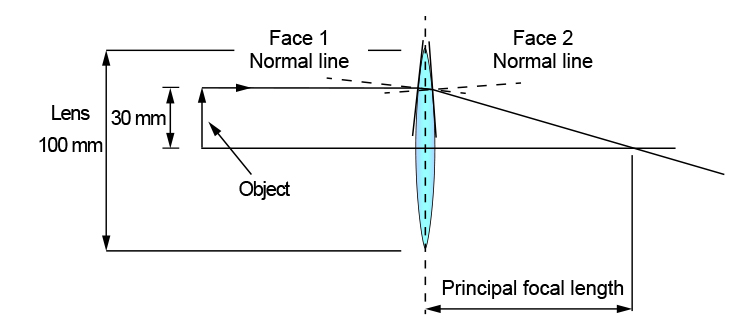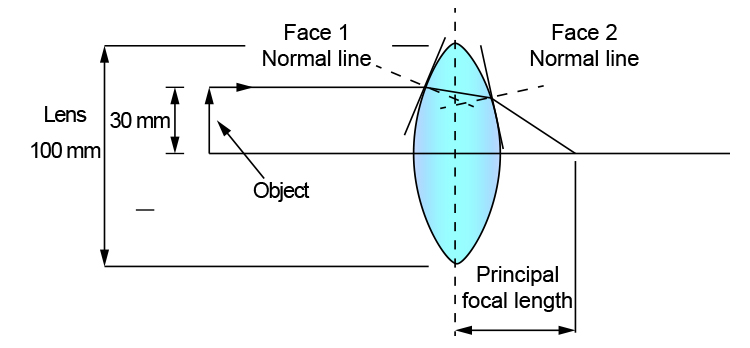# Principal focal length and centre of curvature

If you have two convex lenses of identical shape but made of different materials, a parallel ray of light going through each lens will have a different principal focal length.

However, two convex lenses made out of the same material (same refractive index (n)) will have different principal focal lengths if they have different centres of curvature (radius of spheres). Another way of saying this is, if two lenses of the same material have different shapes - one a flat surface and the other a rounded one, then they will have different principal focal lengths.

Slightly curved lens = Long focal length
Very curved lens = Short focal length

The greater the curvature of a lens the greater the refraction of the ray of light. Which obviously means that the less the curvature of a lens the less the refraction will be.

## Large centre of curvature (slightly curved lens)## Small centre of curvature (very curved lens)